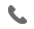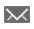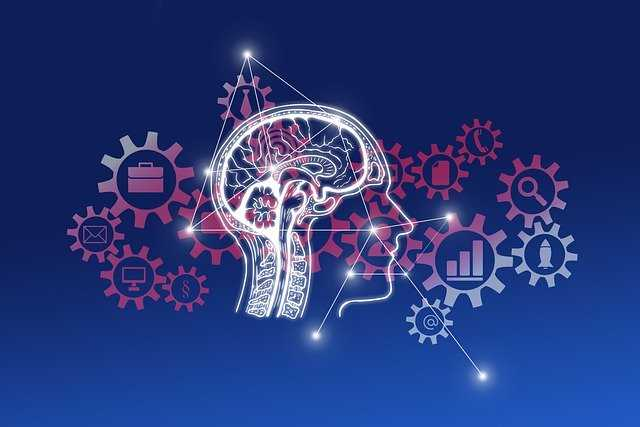•+44 7459 302492info@uplatz.com
• Register
Job Meter = High

### Deep Learning with TensorFlow

25 Hours
Self-paced Training (pre-recorded videos)
USD 17 (GBP 100)
Save 88% Offer ends on 30-Nov-2023234 Learners

Deep Learning is a subfield of machine learning, a powerful set of technique inspired by the structure & function of brain called artificial neural networks. Deep learning is the technology behind driverless cars, Key voice control in consumer device like phones and TVs, used to identified objects from satellites and in medical research, etc.

Neural networks is a set of algorithms, a biological- inspired programming after the human brain, designed to recognise complex patterns and enables a computer to learn from observational data . Neural networks have the ability to model complex relationship in real time. It can generalise learnings from the initial input & their relationship. Neural Networks has lot of capacity to take in inputs and plays a big role in image and character recognition.

In this Deep Learning with TensorFlow course by Uplatz, you will be able to learn the basics and introduction of deep learning and neural networks, multi-layer perception and its parameter, TensorFlow introduction and its open-source software library, Dnns, Cnns and Gpu in deep learning and many more.

-----------------------------------------------------------------------------------------------------------------

# Deep Learning with TensorFlow

Course Details & Curriculum

Module 01 - Introduction to Deep Learning and Neural Networks

1.1 Field of machine learning, its impact on the field of artificial intelligence
1.2 The benefits of machine learning w.r.t. Traditional methodologies
1.3 Deep learning introduction and how it is different from all other machine learning methods
1.4 Classification and regression in supervised learning
1.5 Clustering and association in unsupervised learning, algorithms that are used in these categories
1.6 Introduction to ai and neural networks
1.7 Machine learning concepts
1.8 Supervised learning with neural networks
1.9 Fundamentals of statistics, hypothesis testing, probability distributions, and hidden markov models.

Module 02 - Multi-layered Neural Networks

2.1 Multi-layer network introduction, regularization, deep neural networks
2.2 Multi-layer perceptron
2.3 Overfitting and capacity
2.4 Neural network hyperparameters, logic gates
2.5 Different activation functions used in neural networks, including relu, softmax, sigmoid and hyperbolic functions
2.6 Back propagation, forward propagation, convergence, hyperparameters, and overfitting.

Module 03 - Artificial Neural Networks and Various Methods

3.1 Various methods that are used to train artificial neural networks
3.2 Perceptron learning rule, gradient descent rule, tuning the learning rate, regularization techniques, optimization techniques
3.3 Stochastic process, vanishing gradients, transfer learning, regression techniques,
3.4 Lasso l1 and ridge l2, unsupervised pre-training, xavier initialization.

Module 04 - Deep Learning Libraries

4.1 Understanding how deep learning works
4.2 Activation functions, illustrating perceptron, perceptron training
4.3 multi-layer perceptron, key parameters of perceptron;
4.4 Tensorflow introduction and its open-source software library that is used to design, create and train
4.5 Deep learning models followed by google’s tensor processing unit (tpu) programmable ai
4.6 Python libraries in tensorflow, code basics, variables, constants, placeholders
4.7 Graph visualization, use-case implementation, keras, and more.

Module 05 - Keras API

5.1 Keras high-level neural network for working on top of tensorflow
5.2 Defining complex multi-output models
5.3 Composing models using keras
5.3 Sequential and functional composition, batch normalization
5.4 Deploying keras with tensorboard, and neural network training process customization.

Module 06 - TFLearn API for TensorFlow

6.1 Using tflearn api to implement neural networks
6.2 Defining and composing models, and deploying tensorboard

Module 07 - Dnns (deep neural networks)

7.1 Mapping the human mind with deep neural networks (dnns)
7.2 Several building blocks of artificial neural networks (anns)
7.3 The architecture of dnn and its building blocks
7.4 Reinforcement learning in dnn concepts, various parameters, layers, and optimization algorithms in dnn, and activation functions.

Module 08 - Cnns (convolutional neural networks)

8.1 What is a convolutional neural network?
8.2 Understanding the architecture and use-cases of cnn
8.3‘What is a pooling layer?’ how to visualize using cnn
8.4 How to fine-tune a convolutional neural network
8.5 What is transfer learning?
8.6 Understanding recurrent neural networks, kernel filter, feature maps, and pooling, and deploying convolutional neural networks in tensorflow.

Module 09 - Rnns (recurrent neural networks)

9.1 Introduction to the rnn model
9.2 Use cases of rnn, modeling sequences
9.3 Rnns with back propagation
9.4 Long short-term memory (lstm)
9.5 Recursive neural tensor network theory, the basic rnn cell, unfolded rnn,  dynamic rnn
9.6 Time-series predictions.

Module 10 - Gpu in deep learning

10.1 Gpu’s introduction, how are they different from cpus, the significance of gpus
10.2 Deep learning networks, forward pass and backward pass training techniques
10.3 Gpu constituent with simpler core and concurrent hardware.

Module 11- Autoencoders and restricted boltzmann machine (rbm)

11.1 Introduction to rbm and autoencoders
11.2 Deploying rbm for deep neural networks, using rbm for collaborative filtering
11.3 Autoencoders features and applications of autoencoders.

Module 12 - Deep learning applications

12.1 Image processing
12.2 Natural language processing (nlp) – Speech recognition, and video analytics.

Module 13 - Chatbots

13.1 Automated conversation bots leveraging any of the following descriptive techniques:  Ibm watson, Microsoft’s luis, Open–closed domain bots,
13.2 Generative model, and the sequence to sequence model (lstm).

-----------------------------------------------------------------------------------------------------------------

Job Prospects

----------------------------------------------------------------------------------------------------------

#### Deep Learning with TensorFlow Interview Questions

----------------------------------------------------------------------------------------------------------

Q1. What is Tensorflow?

TensorFlow is a machine learning library created by the Brain Team of Google and made open source in 2015. Basically, Tensorflow is a low-level toolkit for doing complicated math and it offers the users customizability to build experimental learning architectures, to work with them and to turn them into running software.

Q2. What does the latest release of TensorFlow have to offer?

The latest release of TensorFlow is 1.7.0 and is available on www.tensorflow.org. It has been designed with deep learning in mind but applicable to a much wider range of problems.

Q3. What are Tensors?

Tensors are higher dimensional arrays which are used in computer programming to represent a multitude of data in the form of numbers. There are other n-d array libraries available on the internet like Numpy but TensorFlow stands apart from them as it offers methods to create tensor functions and automatically compute derivatives.

Q4. What is a TensorBoard?

TensorBoard, a suit of visualizing tools, is an easy solution to Tensorflow offered by the creators that lets you visualize the graphs, plot quantitative metrics about the graph with additional data like images to pass through it.

Q5. What are the features of TensorFlow?

Tensorflow has APIs for Matlab, and C++ and has a wide language support. With each day passing by, researchers working on making it more better and recently in the latest Tensorflow Summit, tensorflow.js, a javascript library for training and deploying machine learning models has been introduced.

Q6. List a few advantages of TensorFlow?

• It has platform flexibility

• It is easily trainable on CPU as well as GPU for distributed computing.

• TensorFlow has auto differentiation capabilities

• It has advanced support for threads, asynchronous computation, and queue es.

• It is a customizable and open source.

Q7. List a few limitations of Tensorflow.

• Has GPU memory conflicts with Theano if imported in the same scope.

• No support for OpenCL

• Requires prior knowledge of advanced calculus and linear algebra along with a pretty good understanding of machine learning.

Q8. What are TensorFlow servables?

These are the central rudimentary units in TensorFlow Serving.  Objects that clients use to perform the computation are called Servables.

The size of a servable is flexible. A single servable might include anything from a lookup table to a single model to a tuple of inference models.

Q9. What do the TensorFlow managers do?

Tensorflow Managers handle the full lifecycle of Servables, including:

• Serving Servables

Tensorflow Loaders are used for adding algorithms and data backends one of which is tensorflow itself. For example, a loader can be implemented to load, access and unload a new type of servable machine learning model.

Q11. What is deep speech?

Deep Speech developed by Mozilla is a TesnsorFlow implementation motivated by Baidu’s Deep Speech architecture.

Q12. What do you mean by sources in TensorFlow?

Sources are in simple terms, modules that find and provide servables. Each Source provides zero or more servable streams. One Loader is supplied for each servable version it makes available to be loaded.

Q13. How does TensorFlow make use of the python API?

Python is the most recognisable and “the main” language when it comes to TensorFlow and its development. The first language supported by TensorFlow and still supports most of the features. It seems as TensorFlow’s functionality first define in Python and then moved to C++.

Q14. What are the APIs inside the TensorFlow project?

The API’s inside TensorFlow are still Python-based and they have low-level options for its users such as tf.manual or tf.nnrelu which use to build neural network architecture. These APIs also use to aid designing deep neural network having higher levels of abstraction.

Q15. What are the APIs outside TensorFlow project?

• TFLearn: This API shouldn’t be seen as TF Learn, which is TensorFlow’s  tf.contrib.learn. It is a separate Python package.

• TensorLayer: It comes as a separate package and is different from what TensorFlow’s layers API has in its bag.

• Pretty Tensor: It is actually a Google project which offers a fluent interface with chaining.

• Sonnet: It is a project of Google’s DeepMind which features a modular approach.

Q16. How does TensorFlow use the C++ API?

The runtime of TensorFlow is written in C++ and mostly C++ is connected to TensorFlow through header files in tensorflow/cc. C++ API still is in experimental stages of development but Google is committed to working with C++.

Q17. In TensorFlow, what exactly Bias and Variance are? Do you find any similarity between them?

In the learning algorithms, Biases can consider as errors which can result in failure of entire model and can alter the accuracy. Some experts believe these errors are essential to enable leaner’s gain knowledge from a training point of view.

Q18. Can TensorFlow be deployed in container software?

Tensorflow can also use with containerization tools such as docker, for instance, it could use to deploy a sentiment analysis model which uses character level ConvNet networks for text classification.

Q19. What exactly Neural Networks are? What are the types of same you are familiar with?

Neural networks as the name suggests are a network of elemental processing entities that together make a complex form. There can be Artificial Neural Networks and Biological Neural Networks. The use of artificial neural networks is more common as they try to imitate the mechanics of the human brain.

Q20. What are the general advantages of using the Artifical Neural Networks?

They use to find answers to complex problems in a stepwise manner. All the information that a network can receive can easily be in any format. They also make use of real-time operations along with a good tolerance capability.

Q21. What exactly do you know about Recall and Precision?

The other name of Recall is the true positive rate. It is the overall figure of positiveness a model can generally claim. The predictive value which is generally positive in nature is Precision. The difference between the true positive rate and claimed positive rate can be defined with the help of both these options.

Q22. Name some products built using TensorFlow?

TensorFlow built the following products:

Teachable Machine

Nsynth

Handwriting

Giorgio Camthat

Q23. What are some advantages of TensorFlow over other libraries?

Debugging facility, scalability, visualization of data, pipelining and many more.

Q24. How can you make sure that overfitting situation is not arriving with a model you are using?

Users need to make sure that their model is simple and not have any complex statement. Variance takes into the account and the noise eliminates from the model data. Techniques like k-fold and LASSO can also help.

Q25. What exactly do you know about a ROC curve and its working?

ROC or region of convergence used to reflect data rates which classify as true positive and false positive. Represented in the form of graphs, it can use as a proximity to swap operations related to different algorithms.

Q26. In the machine learning context, how useful and reliable Bayes’ theorem is?

Baye’s theorem is useful for determining the probability of an event, obtained by dividing the actual positive rate by the false positive rate. Some of the very complex questions and challenges can solve using a simple approach and eliminated with the help of this theorem.

Q27. What difference do you find in Type I and Type II errors?

Type I error means a false positive value while Type II error means a false negative.

Q28. What would be your strategy to handle a situation indicating an imbalanced dataset?

This usually occurs when a vast set of data keep in a single class. Sampling the dataset again is one of the possible solutions and the other one being the migration of data to parallel classes. The dataset should not be damaged.

Q29. What do you know about supervised and unsupervised machine learning?

Supervised learning consists of labelled data which is not necessarily present in unsupervised learning.

Q30. In machine learning based on TensorFlow, what is more important among the performance or the accuracy of a model?

It depends on the overall experience. Both of them have an equal weightage although accuracy is most important in most of the models.

----------------------------------------------------------------------------------------------------------